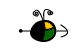It has been determined that the rate of radioactive decay is first order.  We can apply our knowledge of first order kinetics to radioactive decay to determine rate constants, original and remaining amounts of radioisotopes, half-lives of the radioisotopes, and apply this knowledge to the dating of archeological artifacts through a process known as carbon-14 dating.

Calculations Using the First Order Rate Equation:  r = k[N]

Since the rate of radioactive decay is first order we can say:  r = k[N]1, where r is a measurement of the rate of decay, k is the first order rate constant for the isotope, and N is the amount of radioisotope at the moment when the rate is measured.  The rate of decay is often referred to as the activity of the isotope and is often measured in Curies (Ci), one curie = 3.700 x 1010 atoms that decay/second.  By knowing the amount of radioisotope and the activity of the sample, the rate constant can be determined.

Determination of the Rate Constant

Example:  A 1.00 g sample of cobalt-60 (59.92 g/mol) has an activity of 1.1 x 103 Ci.  Determine the rate constant.

• First, we need to convert the 1.00 g of sample into number of atoms of cobalt-60 and to convert the activity into numbers of atoms that decay per second.
(1.00 g Co-60)(1 mol Co-60/59.92 g)(6.022 x 1023 atoms Co-60/mol) = 1.01 x 1022 atoms

(1.1 x 103 Ci)(3.700 x 1010 atoms/s/Ci) = 4.1 x 1013 atoms that decay/sec

• Second, we can then use the first-order rate equation to find the rate constant, k
4.1 x 1013 atoms decaying/sec = k[1.01 x 1022 atoms]

k = 4.1 x 10-9 s-1

Top

Determination of the Activity

Example:  Determine the activity (in Curies) of a 2.00 mg sample of Cobalt-60.

• First, we need to convert the 2.00 mg sample of cobalt-60 into numbers of atoms.
(2.00 mg Co-60)(1 g/1000 mg)(1 mol/59.92 g)(6.022 x 1023 atoms/mol) = 2.01 x 1019 atoms

• Second, we use the rate equation to calculate the activity in atoms/second and then convert the answer into curies.
r = (4.1 x 10-9 s-1)(2.01 x 1019 atoms) = 8.2 x 1010 atoms decay/s
(8.2 x 1010 atoms/s)(1 Ci/3.700 x 1010 atoms/s) = 2.2 Ci

Top

Calculations Using the First-Order Rate Equation:  ln(N/No) = -kt

Using the equation for first-order kinetics, the following equation can be derived:

ln(N/No) = -kt

where "N" is the amount of radioisotope remaining after time "t" has elapsed.  "No" is the initial amount of radioisotope at the beginning of the period, and "k" is the rate constant for the radioisotope being studied.  In this equation, the units of measure for N and No can be in grams, atoms, or moles.  It does not matter as long as they are like measures.  The units of measure for time are dependent upon the unit of measure for the rate constant.  The ratio of "N/No" gives the percentage activity as compared to the activity at time zero.  This equation has a variety of applications.

Determination of the Amount of Radioisotope Remaining after Time "t"

Example:  How much of a  2.00 g sample of radioisotope (k = 0.15 min-1) will remain after 20 minutes?

ln(N/2.00g) = -(0.15 min-1)(20 min)
ln(N/2.00 g) = -0.30
N/2.00g = e-0.30 = 0.741
N = 1.48 g

Top

Determination of the Initial Amount of Radioisotope at the Beginning of Time "t"

Example:  A sample of radioisotope has an activity of 450 microcuries after 2 days.  If the rate constant for the isotope is 0.056 d-1, what was the activity of the sample 2 days ago?

ln(450 uCi/No) = -(0.056 d-1)(2 d)
ln(450 uCi/No) = -0.112
450 uCi/No = e-0.112 = 0.894
No = 503 uCi

Top

Determination of the Rate Constant

Example:  A sample is 70 % as active after 20 hours have elapsed.  Determine the value of the rate constant.

ln(70/100) = -k(20 h)
-0.357 = -k(20 h)
k = 0.018 h-1

Top

Determination of the Time to Decay

Example:  How long will it take a radioisotope to decay to 30% of its original activity if the rate constant for the isotope is 0.055 s-1?

ln(30/100) = -(0.055 s-1)t
-1.20 = -(0.055 s-1)t
t = 22 s

Top

Determination and Use of the Half-Life

Example:  What is the half-life of a radioisotope that has a rate constant of 0.225 d-1?

Half-life (t1/2) is the time for the radioisotope to reach 50% of its original amount.

ln(50/100) = -(0.225 d-1)t1/2
-0.6931 = -(0.225 d-1)t1/2
t1/2 = 3.08 days

Example:  How much of a 60 Ci sample of radioisotope will remain after 10 minutes if the half-life of the radioisotope is 2.75 min?

ln(50/100) = -k(2.75 min)
k = 0.252 min-1

ln(N/60 Ci) = -(0.252 min-1)(10 min)
N = 4.8 Ci

Top

Carbon-14 Dating

Carbon-14 is a radioisotope formed in our atmosphere by the bombardment of nitrogen-14 by cosmic rays.  The amount of carbon-14 in the atomosphere is, on an average, relatively constant.  Plants take in carbon-14 through the process of photosynthesis.  Animals eat the plants so they too have carbon-14 in their tissues.  Carbon-14 is decaying constantly with a half-life of 5720 years.  As long as the organism is alive, the amount of carbon-14 remains relatively constant.  However, when the organism dies, the amount will decrease over time.  By comparing the activity of an archeological artifact to that of a sample of the living organism one can estimate the age of the artifact.

Example:  A sample of wood taken from an ancient tomb had an activity of 7.0 counts per minute (decays per minute).  A similar sample of freshly cut wood of the same type of tree had an activity of 15.3 cpm.  Estimate the age of the wood taken from the tomb.

ln(50/100) = -k(5720 y)
k = 1.212 x 10-4 y-1

ln(7.0 cpm/15.3 cpm) = -(1.212 x 10-4 y-1)t
t = 6982 y